# Spectral semi-invariant

spectral cumulant

One of the characteristics of a stationary stochastic process. Let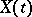,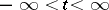, be a real stationary stochastic process for which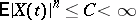. The semi-invariants (cf. Semi-invariant) of this process,are connected with the moments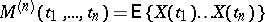by the relations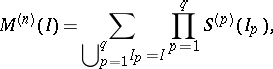whereand the summation is over all partitions ofinto disjoint subsets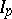. It is said thatif, for all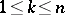, there is a complex measure of bounded variation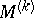onsuch that for all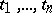,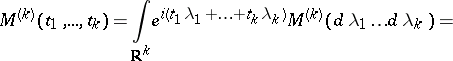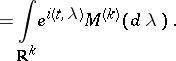A measure, defined on a system of Borel sets, is called a spectral semi-invariant if, for all,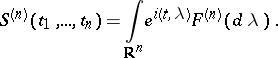The measure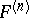exists and has bounded variation if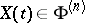. In the case of a stationary process, the semi-invariantsare invariant under translation:and the spectral measuresandare concentrated on the manifold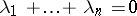. If the measureis absolutely continuous with respect to Lebesgue measure on this manifold, then there is a spectral density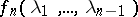of order, defined by the equations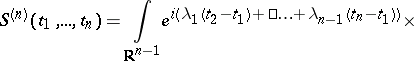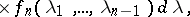for all. In the case of discrete time one must replace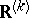in all formulas above by the-dimensional cube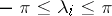,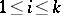.